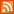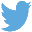Bucaro TecHelpHTTPS Encryption not required because no account numbers orpersonal information is ever requested or accepted by this siteCustom Search# Working With Signed Numbers

Working with signed numbers is easy, it requires only a few easy rules. But if you don't work with signed numbers every day, you could easily forget the rules.

If one of the numbers is zero - Any number plus zero is that number.
If both numbers are positive - Add the numbers, the sum is positive.
If both numbers are negative - Add the numbbers, the sum is negative.
If one number is positive and One is negative - Subtract the smaller number from the larger number.

The sign of the answer, called the sum, is the sign of the largest absolute value number. Absolute value means the number without the sign.)

If a number is positive, we usually leave out the sign, and +0 and -0 are both the same thing, just 0 (zero).

Examples

 46 + 0 = 46 Any number plus zero is that number 27 + 46 = 73 Both numbers are positive - Add the numbers, the sum is positive. -27 + (-46) = -73 Both numbers are negative - Add the numbbers, the sum is negative. 27 + (-46) = -19 One number is positive and one is negative - Subtract the smaller number from the larger number. Here -46 is the largest number. -27 + 46 = 19 One number is positive and one is negative. Here 46 (+46) is the largest number.

Rule for Subtracting Signed Numbers

Change the sign of the number being subtracted, then add using the rules for adding signed numbers.

Examples

 46 - 0 = 46 - (+0) = 46 Whether you add zero, to or subtract zero from a number, the answer is always that number. 0 - 46 = 0 + (-46) = -46 One number is positive and one is negative - Subtract the smaller number from the larger number. Here -46 is the largest number. 27 - 46 = 27 + (-46) = -19 One number is positive and one is negative. The sign of the sum is the sign of the largest (absolute value) number. Here -46 is the largest number. 46 - 27 = 46 + (-27) = 19 One number is positive and one is negative. The sign of the sum is the sign of the largest (absolute value) number. Here 46 (+46) is the largest number.

The subtrahend is the second number in a subtraction, the number to be subtracted. The first number in a subtraction, the minuend is the number from which the subtrahend is to be subtracted.

Multiplying Signed Numbers

If one of the numbers is zero - The answer is always zero.
If both numbers have the same sign - Multiply the numbers, the answer is always positive.
If the Numbers have different signs - Multiply the numbers, the answer is always negative.

Examples

 12(0) = 0 If one of the numbers is zero - The answer is always zero. 0(-9) = 0 If one of the numbers is zero - The answer is always zero. 8(4) = 32 If both numbers have the same sign - Multiply the numbers, the answer is always positive. (-8)(-4) = 32 If both numbers have the same sign - Multiply the numbers, the answer is always positive. 12(-4) = -48 If the Numbers have different signs - Multiply the numbers, the answer is always negative. (-3)10 = -30 If the Numbers have different signs - Multiply the numbers, the answer is always negative.

The multiplicand is a number that is to be multiplied by another number (the multiplier). The multiplier is the number of times the multiplicand gets multiplied. It doesn't matter which comes first, the result, the product is the same either way.

 .menubtn { margin-top:10px; margin-left:50px; width:220px; opacity:1; border-style:solid; border-color:#009000; background-color:#66ff66; transition-property: opacity; transition-duration: 1s; transition-timing-function: ease-out; } .menubtn:hover { opacity:1; background-color:#e5ff23; } Menu - More How To ...RSS FeedFollow @Stephen Bucaro[Site User Agreement] [Privacy Policy] [Site map] [Search This Site] [Contact Form]
Copyright©2001-2019 Bucaro TecHelp 13771 N Fountain Hills Blvd Suite 114-248 Fountain Hills, AZ 85268